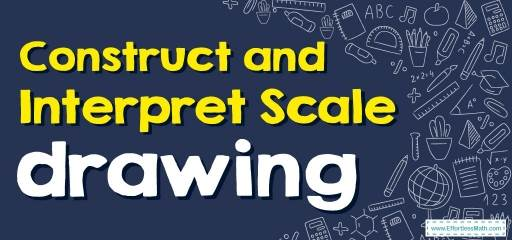# How to Construct and Interpret Scale Drawing? (+FREE Worksheet!)This article will learn how to construct and interpret Scale Drawing in a few simple steps.

## Step by step guide to construct and interpret Scale Drawing

Since it is not always possible to draw the actual size of real objects on paper, we use scale drawings to show them on paper. A scale is a drawing that shows a real object in a way that its size is accurate and has been reduced or enlarged by a certain amount.

The scale is shown as the length in the drawing, matching length on the real thing, and a colon (“:”) between them.

The scale can actually be thought of as a fraction, which is usually the numerator of the fraction is one, and the denominator of that fraction is a whole number. The number at the denominator of the fraction is called the scale number.

The enlargement of the scaled image depends on the difference between the numbers on either side of the ratio or the difference between the numerator and the denominator of the fraction. So, for the 6:1 scale ratio, a 1inch drawing will be 10 inches in real life.

The larger the scale number, the smaller the size of the scaled image on the map.

### Constructing and Interpreting Scale drawing Example 1:

Write the scale of the drawing is $$1cm:9ft$$. find the actual length for a drawing length of $$11cm$$.

Solution: write the scale of the drawing, $$1cm:9ft$$, as $$\frac{1cm}{9ft}$$

Then write a proportion in which each ratio compares centimeters to feet.

$$x$$ represents the actual length. $$\frac{1}{9}=\frac{11}{x}→1(x)=99→x=99ft$$

### Constructing and Interpreting Scale drawing Example 2:

The scale drawing of this building is $$1cm:380ft$$. If the height of the building on paper is $$22cm$$ inches, what is the height of the building in real life?

Solution: set up a proportion like this: $$\frac{height of drawing}{real height}=\frac{1}{380}$$

Do a cross-product by multiplying the numerator of one fraction by the denominator of the other fraction. We get: $$(height of drawing) \times 400=(real height)\times 1$$

Since the height of drawing$$=22$$, we get: $$=22 \times 380=real height \times 1→real height=8,360in$$

### Constructing and Interpreting Scale drawing Example 3:

A particular map shows a scale of $$1:7000$$. What is the actual distance if the map distance is $$14cm$$?

Solution: write the scale of the drawing, $$1:7000$$, as $$\frac{1}{7000}$$

Then write a proportion in which each ratio compares centimeters to feet.

$$x$$ represents the actual length. $$\frac{1}{7000}=\frac{14}{x}→1(x)=98,000→x=98,000cm=980m$$

### Constructing and Interpreting Scale drawing Example 4:

A rectangle yard has a length of $$45feet$$ and a width of $$36feet$$. Make a scale drawing using a scale of $$1inch: 9feet$$.

Solution: Solve the proportion to find $$x$$:

$$\frac{1in}{9ft}=\frac{x}{45ft}→\frac{1in\times 45ft}{9ft}=x$$

So, $$x=1in\times \frac{45ft}{9ft}=1in\times 5=5in$$

## Exercises for Constructing and Interpreting Scale drawing

1. Write the scale $$12mm$$ to $$1m$$ in ratio form.
2. in a scale drawing, the width of a sofa is $$7cm$$. The actual width of the sofa is $$140cm$$. what is the scale of the drawing?
3. A map has a scale of $$1cm:15mile$$. If two cities are $$5$$ apart on the map, how far are they actually apart?
4. A particular map shows a scale of $$1cm:6km$$. what would the map distance(in cm) be if the actual distance is $$36km$$?
1. $$3mm:250mm$$
2. $$20$$
3. $$75mile$$
4. $$6cm$$

### What people say about "How to Construct and Interpret Scale Drawing? (+FREE Worksheet!) - Effortless Math: We Help Students Learn to LOVE Mathematics"?

No one replied yet.

X
30% OFF

Limited time only!

Save Over 30%

SAVE $5 It was$16.99 now it is \$11.99